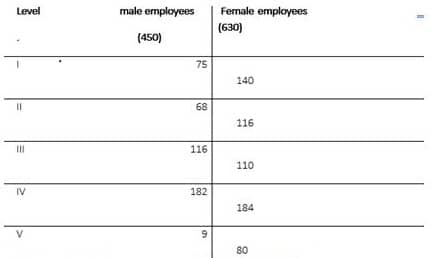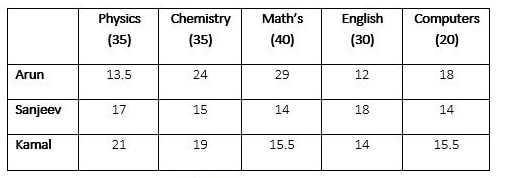Aptitude Questions – Data Interpretation (Caselet) Set 91

Hello Aspirants.

Welcome to Online Maths in AffairsCloud.com. Here we are creating question sample in Data Interpretation, which is common for all the IBPS, SBI, RBI, IPPB, LIC and other competitive exams. We have included Some questions that are repeatedly asked in exams !!

Data Interpretation

Directions (1-5): The ratio of male to female employees in an organization is 5 : 7. All employees of the organization work at different levels. (Level- I, II, III, IV, V). 16 2/3% of the male employees work at level I. The difference between male employees working at level II and male employees working at level IV is 114, while the sum of the same is 250. (male employees at level II < male employees at level IV). 9 male employees work at level V, which is 2% of the total number of male employees. remaining male employees work at level III. 22 2/9% of the female employees work at level I. The no. Female employees working at level II is 6 more than the no. of female employees working at level III. The number of female employees working at level IV is 2 more than the number of male employees at the same level. The number of female employees working at level V is 57 1/7% of the female employees working at level I.

1. Find the total number of employees working on level II.
1) 176
2) 184
3) 188
4) 192
5) None of these
Explanation :Required number = 68 + 116 = 184

2. Female employees working on level V constitutes what percent of total number of employees in the organization?
1) 5 1/9%
2) 5 1/3%
3) 5 5/9%
4) 7 11/27%
5) None of these
Explanation :
Required percentage = (80/1080)×100=7 (11/27)%

3. What is the respective ratio of male employees working at level I to the female employees working at level V?
1) 15 : 16
2) 14 : 15
3) 15 : 17
4) 17 : 19
5) None of these
Explanation :
Required ratio = 75/80=15∶16

4. What is the total number of employees working at level I, level II and level III?
1) 325
2) 425
3) 475
4) 525
5) None of these
Answer – 5) None of these
Explanation :
Total no. of employees at level I, level II and level III
= 75 + 68+ 116 + 140 + 116 + 110
= 625

5. The number of female employees of which level is equal to the number of male employees of level III?
1) level I
2) level II
3) level III
4) level IV
5) level V
Explanation :
number of female employees of level 2 is equal to the number of male employees of level III.

Directions (6-10): Given below is the information regarding to the result of 3 students Arun, Sanjeev, Kamal in sessional exams of class 12th. Read it carefully and answer the following questions: –

There are total 5 subjects i.e. Physics, Chemistry, Maths, English, and computers each carrying different maximum marks. Physics and Chemistry both carries equal maximum marks i.e. 35. Math carries maximum marks 5 more than Physics and 10 more than English. Total of maximum marks of the 5 subjects is 160. Kamal scored 60% in Physics, while Sanjeev scored 48 4/7% in the same subject. Arun scored only 13.5 marks in Physics. Arun scored 24 marks in Chemistry which is 60% more than the marks scored by Sanjeev in the same subject. The total of the marks of 3 students in chemistry is 58, Kamal scored 15.5 marks in Maths, while Sanjeev scored 35% in the same subject and Arun scored highest in maths with 29 marks. Arun scored 40% in English which is 33 1/3% less than the marks of Sanjeev in the same subject. Score of Kamal in English is 14 marks. The sum of marks of Arun and Sanjeev in computers is 32 while the ratio of the same is 9 : 7. Kamal scored 77.5% marks in computers.

1. What is the average marks scored by the 3 students in English? (round off to nearest integer)
1) 11
2) 14
3) 18
4) 15
5) None of these
Explanation :Required average=(12+18+14)/3=15

2. Find the difference between total marks scored by Arun in all subjects and the total marks scored by Sanjeev in all subjects together.
1) 12
2) 18.5
3) 17
4) 21
5) None of these
Explanation :
Required difference =96.5-78=18.5

3. Calculate the percentage of marks obtained by Kamal in the sessional exams.
1) 50.5%
2) 52.25%
3) 53.125%
4) 53.75%
5) None of these
Explanation :
required percentage =(85/160)×100=53.125%

4. Marks of Sanjeev in English and Math’s in what percent more or less than by marks of all the 3 students in computers?(round off to 2 decimal places).
1) 32.63%
2) 33.33%
3) 35.63%
4) 36.63%
5) 38.63%
Explanation :
required percentage =(47.5 – 32)/47.5×100=32.63%

5. If the passing marks in each sessional are 40%, then total number of compartments of all students together?
1) 1
2) 2
3) 3
4) 4
5) 5